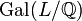# Number of irreducible representations over rationals equals number of equivalence classes under rational conjugacy

## Statement

Suppose$G$ is a finite group. Then, the following numbers are equal:

1. The number of irreducible linear representations of$G$ over the field of rational numbers.
2. The number of linear representations of$G$ over$\mathbb{C}$ with rational character values and for which no proper nonzero subrepresentation has rational character values.
3. The number of equivalence classes in$G$ under rational conjugacy.
4. The number of conjugacy classes of cyclic subgroups in$G$.

## Caveats

The number of irreducible representations over rationals is not the same as the number of irreducible representations over the complex numbers that can be realized over the rationals. The latter number is either smaller or equal, and it is equal when the group is a rational group, which means that any two elements generating the same cyclic subgroup are conjugate.

Also, although the counts in (1) and (2) are equal, it is possible for a rational character to arise from an irreducible representation over the complex numbers that is not realized over the rationals. However, some multiple of that representation can be realized over the rationals. This explains the equality of counts in (1) and (2). The smallest multiple used is termed the Schur index.

## Facts used

1. Sufficiently large implies splitting
2. Orbits of irreducible representations of extension field under Galois group are in bijection with irreducible representations of base field
3. Orbit-counting theorem (specifically, this is used to show that the character, or number of fixed points for each element, of a permutation representation determines its number of orbits).
4. Application of Brauer's permutation lemma to Galois automorphism on conjugacy classes and irreducible representations (follows in turn from Brauer's permutation lemma): Suppose$G$ is a finite group and$r$ is an integer relatively prime to the order of$G$. Suppose$K$ is a field and$L$ is a splitting field of$G$ of the form$K(\zeta)$ where$\zeta$ is a primitive$d^{th}$ root of unity, with$d$ also relatively prime to$r$ (in fact, we can arrange$d$ to divide the order of$G$ because sufficiently large implies splitting). Suppose there is a Galois automorphism of$L/K$ that sends$\zeta$ to$\zeta^r$. Consider the following two permutations:
• The permutation on the set of conjugacy classes of$G$, denoted$C(G)$, induced by the mapping$g \mapsto g^r$.
• The permutation on the set of irreducible representations of$G$ over$L$, denoted$I(G)$, induced by the Galois automorphism of$L$ that sends$\zeta$ to$\zeta^r$.

Then, these two permutations have the same cycle type. In particular, they have the same number of cycles, and the same number of fixed points, as each other.

## Proof

This proof uses a tabular format for presentation. Provide feedback on tabular proof formats in a survey (opens in new window/tab) | Learn more about tabular proof formats|View all pages on facts with proofs in tabular format

Given: A finite group$G$.

To prove: The number of irreducible representations of$G$ over the field of rational numbers$\mathbb{Q}$ (note: these need not be absolutely irreducible representations) equals the number of equivalence classes in$G$ under rational conjugacy (which can also be described as the number of conjugacy classes of cyclic subgroups).

Proof: As in the statement of Fact (2), we denote by$C(G)$ the set of conjugacy classes of$G$ and by$R(G)$ the set of irreducible representations of$G$ over a splitting field of characteristic zero.

No. Assertion/construction Facts used Given data used Previous steps used Explanation
1 Let$L$ be the cyclotomic extension$\mathbb{Q}(\zeta)$ where$\zeta$ is a primitive$k^{th}$ root of unity for$k$ the exponent of$G$. Then,$L$ is a splitting field for$G$ in characteristic zero and hence the irreducible representations of$G$ over$\mathbb{C}$ are all realized in$L$. Fact (1)$G$ is a finite group Fact-direct
2 Under the action of the group$\operatorname{Gal}(L/\mathbb{Q})$, the set of orbits in$R(G)$ is in bijection with the set of irreducible representations of$G$ over$\mathbb{Q}$. Fact (2) Apply Fact (2) to the extension$L$ over$\mathbb{Q}$. Note that since it is a cyclotomic extension, it is automatically Galois.
3 We get an action of the group$\operatorname{Gal}(L/\mathbb{Q})$ on$C(G)$ induced as follows: for any element of$\operatorname{Gal}(L/\mathbb{Q})$ that sends$\zeta$ to$\zeta^r$, the induced permutation on$C(G)$ sends the conjugacy class of$g$ to the conjugacy class of$g^r$.
4 Under the action described in Step (3) for the group$\operatorname{Gal}(L/\mathbb{Q})$ on$C(G)$, the set of orbits in$C(G)$ is in bijection with the set of equivalence classes under rational conjugacy in$G$
5 For every element$\sigma \in \operatorname{Gal}(L/\mathbb{Q})$, the cycle type of the permutation induced by$\sigma$ on$R(G)$ is the same as the cycle type of the permutation induced on$C(G)$ as described in Step (3) Fact (4) (Brauer's permutation lemma)
6 For every element$\sigma \in \operatorname{Gal}(L/\mathbb{Q})$, the number of fixed points of the permutation induced by$\sigma$ on$R(G)$ is the same as the number of fixed points of the permutation induced on$C(G)$ as described in Step (3) Step (5) direct
7 The number of orbits of$R(G)$ under the action of$\operatorname{Gal}(L/\mathbb{Q})$ equals the number of orbits of$C(G)$ under the action of$\operatorname{Gal}(L/\mathbb{Q})$. Fact (3) (orbit-counting theorem, also called Burnside's lemma) Step (6) [SHOW MORE]
8 The number of irreducible representations over rationals equals the number of equivalence classes under rational conjugacy Steps (2), (4), (7) [SHOW MORE]